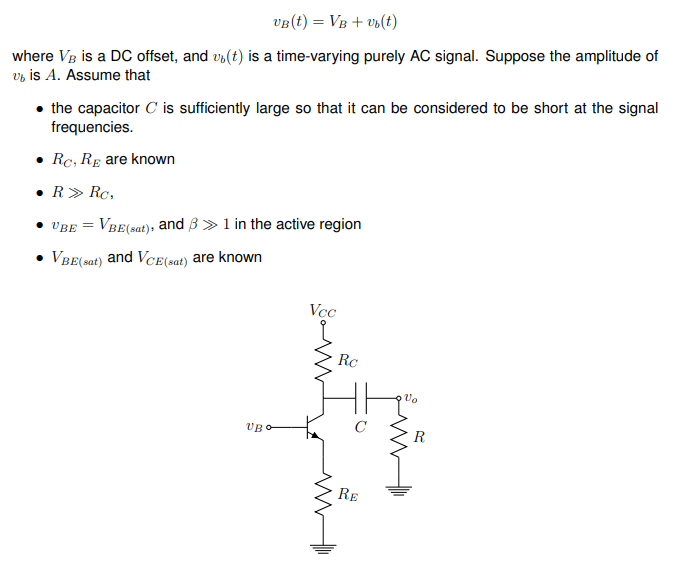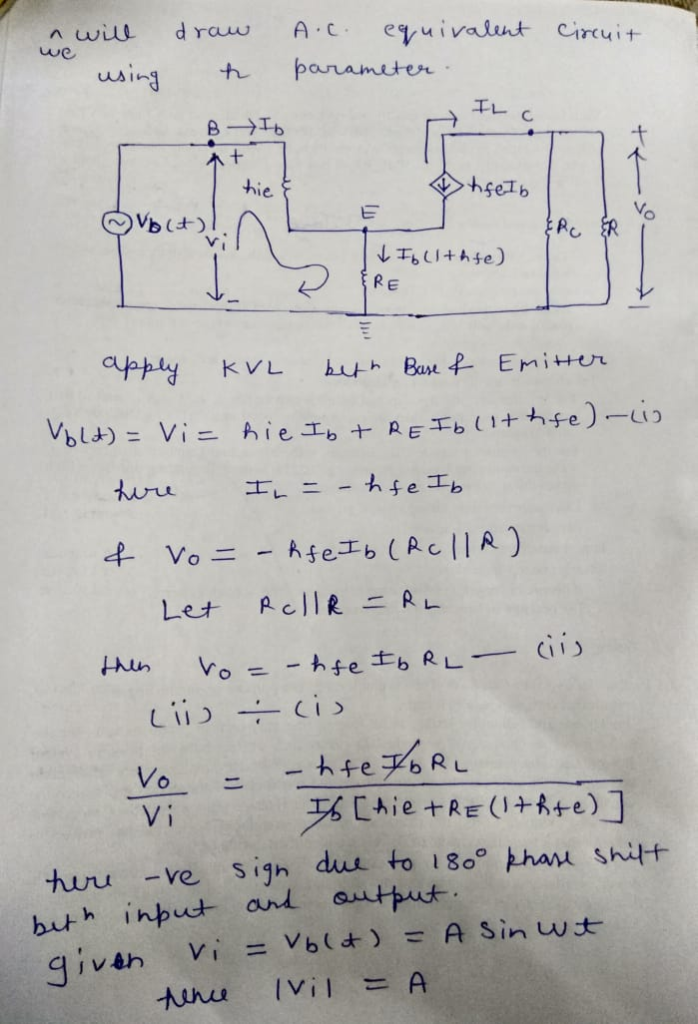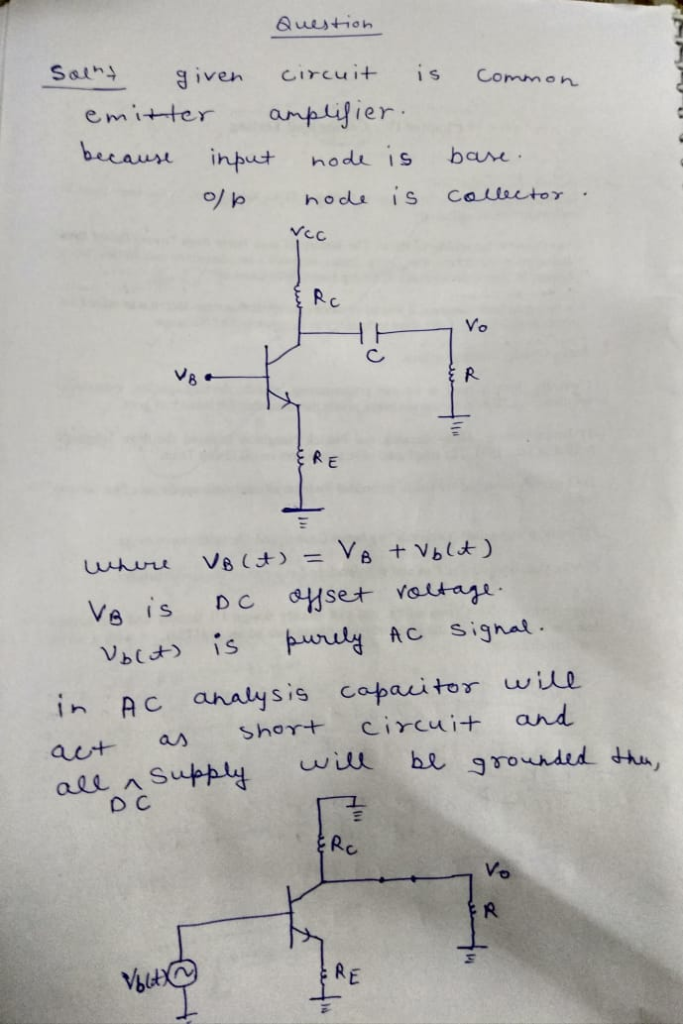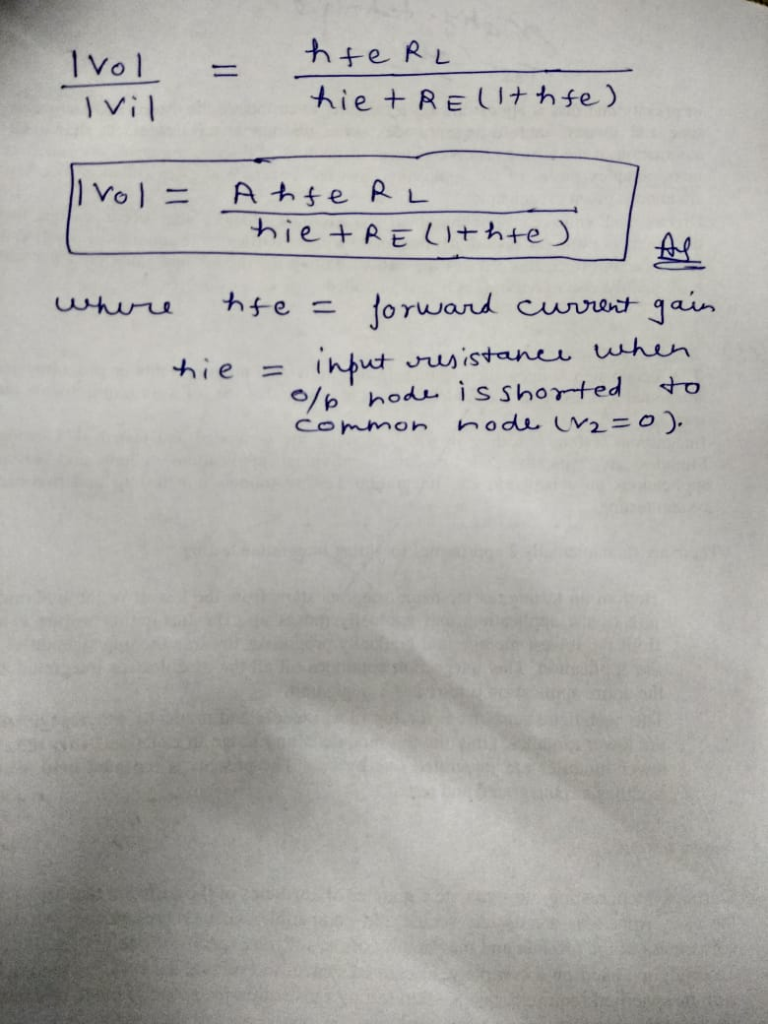Homework Help Question & Answers

In the amplifier circuit in Figure 1 assume further that the transistor remains in the active region at all times. Express the amplitude of "vo" in terms of A and the circuit parameters. Plea...In the amplifier circuit in Figure 1 assume further that the transistor remains in the active region at all times. Express the amplitude of "vo" in terms of A and the circuit parameters. Please express algebraically.

where Ve is a DC offset, and vb(t) is a time-varying purely AC signal. Suppose the amplitude of vb is A. Assume that the capacitor C is sufficiently large so that it can be considered to be short at the signal frequencies. . Rc, RE are known VBE VBE(eat), and B1 n the active region BE(eat) and VcE(eat) are known RcAdd Answer of: In the amplifier circuit in Figure 1 assume further that the transistor remains in the active region at all times. Express the amplitude of "vo" in terms of A and the circuit parameters. Plea...
More Homework Help Questions Additional questions in this topic.

• Assume that the transistor parameters are those for the 2N7000 NMOS transistors in your parts kit. That is: VTN = 2 V, KN = 0.05 A/V2 Draw the bode magnitude and bode phase plot See Figure 2. Assume...

Need Online Homework Help?

Get FREE EXPERT Answers
WITHIN MINUTES
Related Questions